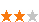您可以捐助，支持我们的公益事业。 1元 10元 50元 认证码：必填求知 文章 文库 Lib 视频 iPerson 课程 认证 咨询 工具 讲座 Modeler Code要资料订阅捐助1822 次浏览     评价： 好 中 差

Wrold是存储N体状态的一个类。我们的模拟将演化一系列时间步长下的状态。

 `class World(object): """World is a structure that holds the state of N bodies and additional variables. threads : (int) The number of threads to use for multithreaded implementations. dt : (float) The time-step. STATE OF THE WORLD: N : (int) The number of bodies in the simulation. m : (1D ndarray) The mass of each body. r : (2D ndarray) The position of each body. v : (2D ndarray) The velocity of each body. F : (2D ndarray) The force on each body. TEMPORARY VARIABLES: Ft : (3D ndarray) A 2D force array for each thread's local storage. s : (2D ndarray) The vectors from one body to all others. s3 : (1D ndarray) The norm of each s vector. NOTE: Ft is used by parallel algorithms for thread-local storage. s and s3 are only used by the Python implementation. """ def __init__(self, N, threads=1, m_min=1, m_max=30.0, r_max=50.0, v_max=4.0, dt=1e-3): self.threads = threads self.N = N self.m = np.random.uniform(m_min, m_max, N) self.r = np.random.uniform(-r_max, r_max, (N, 2)) self.v = np.random.uniform(-v_max, v_max, (N, 2)) self.F = np.zeros_like(self.r) self.Ft = np.zeros((threads, N, 2)) self.s = np.zeros_like(self.r) self.s3 = np.zeros_like(self.m) self.dt = dt`

1. 合力F，每个体上的合力根据所有其他体的计算。

2. 速度v，由于力的作用每个体的速度被改变。

3. 位置R，由于速度每个体的位置被改变。

 `#define r(x0, x1) (*(npy_float64*)((PyArray_DATA(py_r) + \ (x0) * PyArray_STRIDES(py_r) + \ (x1) * PyArray_STRIDES(py_r))))`

 `static PyObject *evolve(PyObject *self, PyObject *args) { // Declare variables. npy_int64 N, threads, steps, step, i, xi, yi; npy_float64 dt; PyArrayObject *py_m, *py_r, *py_v, *py_F; npy_float64 *m, *r, *v, *F; // Parse arguments. if (!PyArg_ParseTuple(args, "ldllO!O!O!O!", &threads, &dt, &steps, &N, &PyArray_Type, &py_m, &PyArray_Type, &py_r, &PyArray_Type, &py_v, &PyArray_Type, &py_F)) { return NULL; } // Get underlying arrays from numpy arrays. m = (npy_float64*)PyArray_DATA(py_m); r = (npy_float64*)PyArray_DATA(py_r); v = (npy_float64*)PyArray_DATA(py_v); F = (npy_float64*)PyArray_DATA(py_F); // Evolve the world. for(step = 0; step < steps; ++step) { compute_F(N, m, r, F); for(i = 0; i < N; ++i) { // Compute offsets into arrays. xi = 2 * i; yi = xi + 1; v[xi] += F[xi] * dt / m[i]; v[yi] += F[yi] * dt / m[i]; r[xi] += v[xi] * dt; r[yi] += v[yi] * dt; } } Py_RETURN_NONE;}`

 `static inline void compute_F(npy_int64 N, npy_float64 *m, npy_float64 *r, npy_float64 *F) { npy_int64 i, j, xi, yi, xj, yj; npy_float64 sx, sy, Fx, Fy, s3, tmp; // Set all forces to zero. for(i = 0; i < N; ++i) { F[2*i] = F[2*i + 1] = 0; } // Compute forces between pairs of bodies. for(i = 0; i < N; ++i) { xi = 2*i; yi = xi + 1; for(j = i + 1; j < N; ++j) { xj = 2*j; yj = xj + 1; sx = r[xj] - r[xi]; sy = r[yj] - r[yi]; s3 = sqrt(sx*sx + sy*sy); s3 *= s3*s3; tmp = m[i] * m[j] / s3; Fx = tmp * sx; Fy = tmp * sy; F[xi] += Fx; F[yi] += Fy; F[xj] -= Fx; F[yj] -= Fy; } }}`

x86内部

 `static PyObject *evolve(PyObject *self, PyObject *args) { // Variable declarations. npy_int64 N, threads, steps, step, i; npy_float64 dt; PyArrayObject *py_m, *py_r, *py_v, *py_F; npy_float64 *m; __m128d *r, *v, *F; // Parse arguments. if (!PyArg_ParseTuple(args, "ldllO!O!O!O!", &threads, &dt, &steps, &N, &PyArray_Type, &py_m, &PyArray_Type, &py_r, &PyArray_Type, &py_v, &PyArray_Type, &py_F)) { return NULL; } // Get underlying arrays from numpy arrays. m = (npy_float64*)PyArray_DATA(py_m); r = (__m128d*)PyArray_DATA(py_r); v = (__m128d*)PyArray_DATA(py_v); F = (__m128d*)PyArray_DATA(py_F); // Evolve the world. for(step = 0; step < steps; ++step) { compute_F(N, m, r, F); for(i = 0; i < N; ++i) { v[i] += F[i] * dt / m[i]; r[i] += v[i] * dt; } } Py_RETURN_NONE;}`

 `static inline void compute_F(npy_int64 N, npy_float64 *m, __m128d *r, __m128d *F) { npy_int64 i, j; __m128d s, s2, tmp; npy_float64 s3; // Set all forces to zero. for(i = 0; i < N; ++i) { F[i] = _mm_set1_pd(0); } // Compute forces between pairs of bodies. for(i = 0; i < N; ++i) { for(j = i + 1; j < N; ++j) { s = r[j] - r[i]; s2 = s * s; s3 = sqrt(s2 + s2); s3 *= s3 * s3; tmp = s * m[i] * m[j] / s3; F[i] += tmp; F[j] -= tmp; } }}`1822 次浏览     评价： 好 中 差订阅捐助
 相关文章 深度解析：清理烂代码 如何编写出拥抱变化的代码 重构-使代码更简洁优美 团队项目开发"编码规范"系列文章
 相关文档 重构-改善既有代码的设计 软件重构v2 代码整洁之道 高质量编程规范
 相关课程 基于HTML5客户端、Web端的应用开发 HTML 5+CSS 开发 嵌入式C高质量编程 C++高级编程讲座 前端开发框架uni-app 主讲：苏雯斐 时间：2020年8月15日实录 企业架构师的能力模型 主讲：俎涛

 WEB应用程序UI模版代码编写 C# 编码规范和编程好习惯 什么是防御性编程 善于防守-健壮代码的防御性 Visual C++编程命名规则 JavaScript程序编码规范

 设计模式原理与应用 从需求过渡到设计 软件设计原理与实践 如何编写高质量代码 单元测试、重构及持续集成 软件开发过程指南

 某全球知名通信公司 代码整洁 横河电机 如何编写高质量代码 某知名金融软件服务商 代码评审 东软集团 代码重构 某金融软件服务商 技术文档 中达电通 设计模式原理与实践 法国电信 技术文档编写与管理